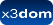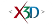X3D: 3.3
Status:
experimental

➨ Fields

### HTML Encoding and Default Values⤴

``` <IndexedQuadSet attrib='x3dom.nodeTypes.X3DVertexAttributeNode' ccw='true' color='x3dom.nodeTypes.X3DColorNode' colorPerVertex='true' coord='x3dom.nodeTypes.X3DCoordinateNode' index='[]' lit='true' metadata='X3DMetadataObject' normal='x3dom.nodeTypes.Normal' normalPerVertex='true' normalUpdateMode=''fast'' solid='true' texCoord='x3dom.nodeTypes.X3DTextureCoordinateNode' useGeoCache='true' ></IndexedQuadSet> ```

### Fields⤴

These are the X3D / X3DOM fields of this node. Values should usually be received / set as strings via DOM functions (i.e., using `setAttribute("myFieldName", "myFieldValue")` and `getAttribute("myFieldName")`).
Name Type Default Value Range Inheritance Standard Description
attrib MFNode Shaders/X3DVertexAttributeNode Rendering/X3DComposedGeometryNodeIf the attrib field is not empty it shall contain a list of per-vertex attribute information for programmable shaders.
ccw SFBool true Rendering/X3DGeometryNodeThe ccw field defines the ordering of the vertex coordinates of the geometry with respect to user-given or automatically generated normal vectors used in the lighting model equations.
color SFNode Rendering/X3DColorNode Rendering/X3DComposedGeometryNodeIf the color field is NULL, the geometry shall be rendered normally using the Material and texture defined in the Appearance node.
colorPerVertex SFBool true Rendering/X3DComposedGeometryNodeIf colorPerVertex is FALSE, colours are applied to each face. If colorPerVertex is true, colours are applied to each vertex.
coord SFNode Rendering/X3DCoordinateNode Rendering/X3DComposedGeometryNodeContains a Coordinate node.
index MFInt32 []IndexedQuadSet uses the indices in its index field to specify the vertices of each quad from the coord field.
lit SFBool true Rendering/X3DGeometryNodeSpecifies whether this geometry should be rendered with or without lighting.
metadata SFNode X3DMetadataObject Core/X3DNodeField to add metadata information
normal SFNode Rendering/Normal Rendering/X3DComposedGeometryNodeIf the normal field is not NULL, it shall contain a Normal node whose normals are applied to the vertices or faces of the X3DComposedGeometryNode in a manner exactly equivalent to that described above for applying colours to vertices/faces (where normalPerVertex corresponds to colorPerVertex and normalIndex corresponds to colorIndex). If the normal field is NULL, the browser shall automatically generate normals in accordance with the node's definition. If the node does not define a behaviour, the default is to generate an averaged normal for all faces that share that vertex.
normalPerVertex SFBool true Rendering/X3DComposedGeometryNodeIf normalPerVertex is FALSE, normals are applied to each face. If normalPerVertex is true, normals are applied to each vertex.
normalUpdateMode SFString 'fast' Rendering/X3DComposedGeometryNodesolid SFBool true Rendering/X3DGeometryNodeSpecifies whether backface-culling is used. If solid is TRUE only front-faces are drawn.
texCoord SFNode Texturing/X3DTextureCoordinateNode Rendering/X3DComposedGeometryNodeIf the texCoord field is not NULL, it shall contain a TextureCoordinate node.
useGeoCache SFBool true Rendering/X3DGeometryNodeMost geo primitives use geo cache and others might later on, but one should be able to disable cache per geometry node. Defaults to global useGeoCache setting parameter.